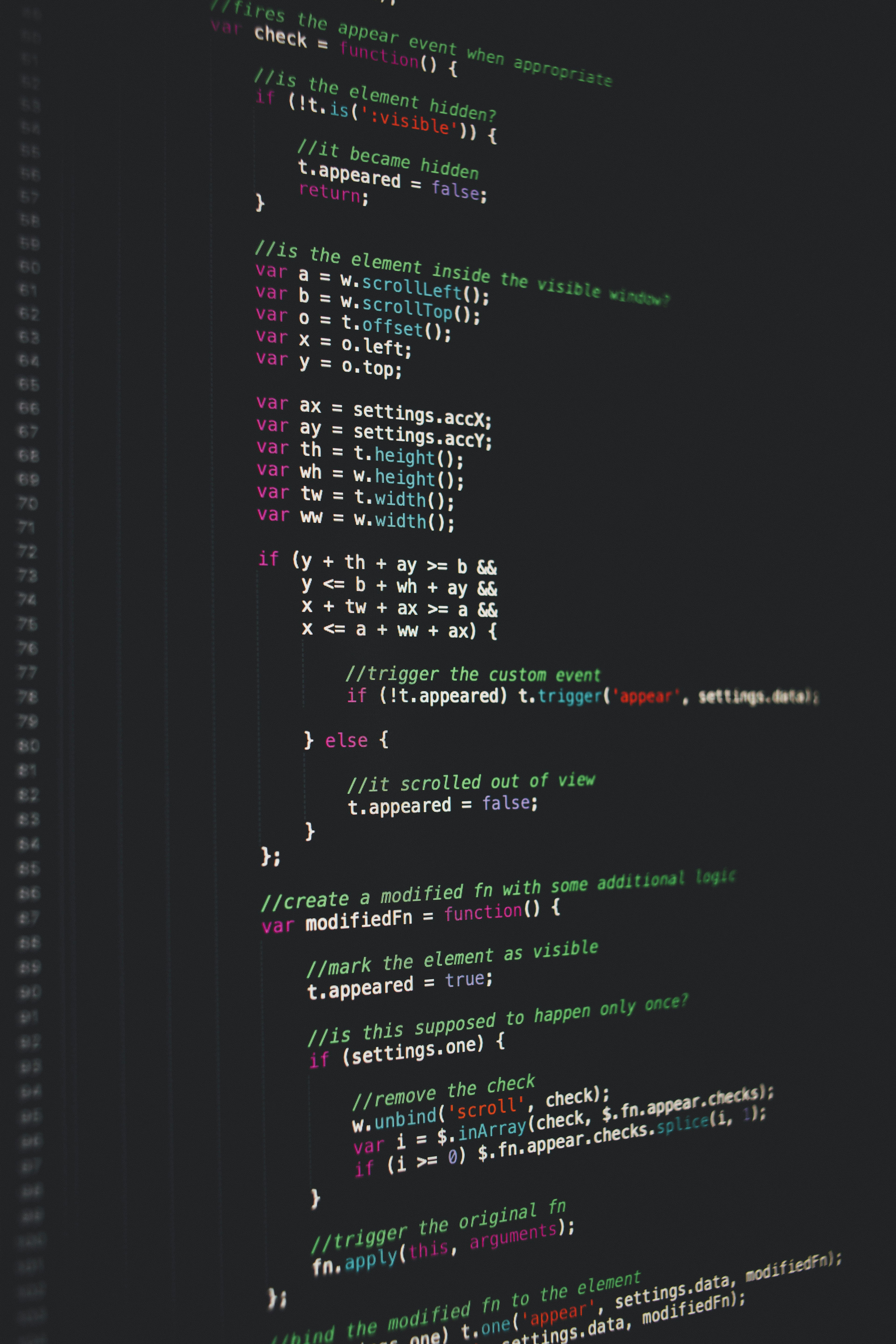Hackerrank C++ Class Template Specialization Solution

# Hackerrank C++ Class Template Specialization SolutionYou are given a main function which reads the enumeration values for two different types as input, then prints out the corresponding  enumeration names. Write a class template that can provide the names of the enumeration values for both types. If the enumeration value is not valid, then print unknown.

Input Format.

The first line contains , the number of test cases.
Each of the  subsequent lines contains two space-separated integers. The first integer is a color value, , and the second integer is a fruit value, .

Constraints.

Output Format.

The locked stub code in your editor prints  lines containing the color name and the fruit name corresponding to the input enumeration index.

Sample Input

2
1 0
3 3


Sample Output

green apple
unknown unknown


Explanation.

Since , there are two lines of output.

1. The two input index values,  and , correspond to green in the color enumeration and apple in the fruit enumeration. Thus, we print green apple.
2. The two input values,  and , are outside of the range of our enums. Thus, we print unknown unknown.

### Solution in cpp

Approach 1.

// Define specializations for the Traits class template here.
template <> struct Traits<Fruit> {
static string name(int i) {
static const string s = {"apple", "orange", "pear"};
if(i>=0 && i<3) return s[i];
return "unknown";
}
};

template <> struct Traits<Color> {
static string name(int i) {
static const string s = {"red", "green", "orange"};
if(i>=0 && i<3) return s[i];
return "unknown";
}
};

Approach 2.

template <>
struct Traits<Fruit> {
static string name(int idx) {
static const string fname[] = { "apple", "orange", "pear" };
if (idx < 0 || idx > 2)
return "unknown";
return fname[idx];
}
};

template <>
struct Traits<Color> {
static string name(int idx) {
static const string cname[] = { "red", "green", "orange" };
if (idx < 0 || idx > 2)
return "unknown";
return cname[idx];
}
};

Approach 3.

#include <vector>
template <>
struct Traits<Fruit> {
static string name(int index) {
static vector<string> const names {"apple", "orange", "pear"};
if (index < 0 || index >= names.size())
return "unknown";
return names[index];
}
};

template <>
struct Traits<Color> {
static string name(int index) {
static vector<string> const names {"red", "green", "orange"};
if (index < 0 || index >= names.size())
return "unknown";
return names[index];
}
};